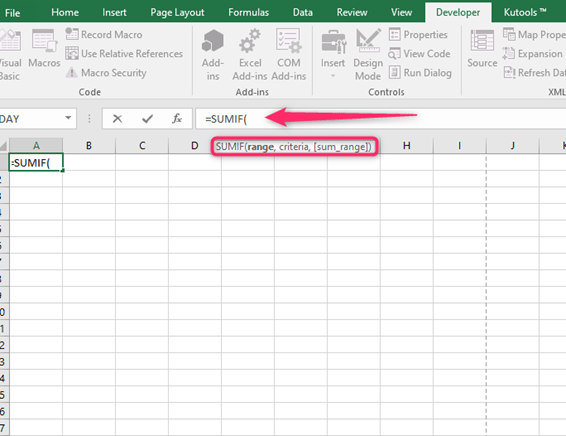# In Excel, How to sum only positive or Negative numbers – Formula

When working with Excel datasheets, you may often need to conditional add the data. You may need to sum only the positive numbers or sum only the negative numbers in Excel. It can easily be done using the built-in formulas within Excel. Below, we guide you on how to do this.

Let's look at the steps you can follow to sum only positive or negative numbers in Excel with formulas

## How to use the SUMIF function to sum only positive or negative numbers in a range

Suppose you have values in column B of your excel sheet, and your task is to sum positive only or negative only values. To achieve this, you will use one of the formulas below

For positives only =SUMIF (\$B: \$B, ">0")

For negatives only =SUMIF (\$B: \$B, "<0")

The SUMIF function in Excel sum the numbers in the range of cells that meet a single criterion you specify. The syntax of the SUMIF function is as follows;

=SUMIF (range, criteria, [sum_range])Where;

• The range is the criteria range of cells that contain the data you want the function to work on. You focus on adding only the values that meet the criteria.
• Criteria are the condition you want to be satisfied to include a cell in the sum. Remember always to use an operator within double-quotes.
• Sum_range is the optional parameter. You do not need this one when finding the sum of positive numbers.

Steps to follow, to sum up, positive values

Assume you have values that range B3: B12. The SUMIF function will be =SUMIF (B3: B12. ">0")

To sum up the positive numbers in this datasheet,

1. Click on the cell where you want your result to be displayed. In our case, it will be cell B13.2. In this cell, type in the equal to sign (=), followed by the SUMIF and an opening bracket, i.e., =SUMIF(3. Next, you will select the range of values you want to find. In our example, you will select cells B3 to B12.4. After doing so, a reference to cells B3: B12 should appear after the open bracket in cell B13

5. Next, insert a comma (,) followed by the condition >0, which should be within double quotes, i.e., ">0."

6. Insert a closing bracket after the condition. Your complete formula in cell B13 will be =SUMIF (B3: B12, ">0").7. Lastly, press the Return or Enter key. You will be able to see the resulting sum of positive numbers in cell B13.

If you want to sum all the negative values in cells B3 to B12, you can use the same formula with minor changes. It will be as below;

=SUMIF (B3: B12, "<0")To get the values in cell B13, you follow the above steps. Remember to use the condition "<0" in step 5.

Note;

SUMIF function offers Excel users an additional advantage. After the comma, you can add one or more conditions. For instance, suppose you have a data set, and you want to find all positive numbers greater than 100 within a range. You can use the formula below

=SUMIF (B3: B12, ">100", B3: B12)